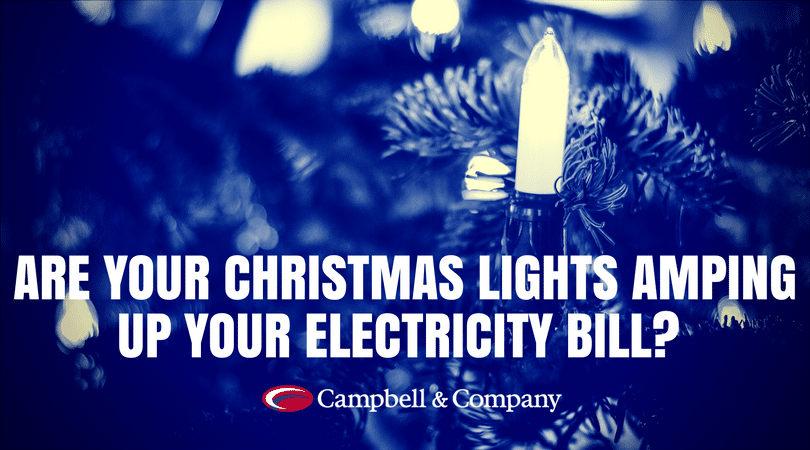###### Fats, Oil, & Grease How Bad Can It Be?
November 21, 2017###### New Year Resolutions for your home
January 8, 2018Everyone wants to save money during the holidays. If you want to save money with your Christmas lights display, it is helpful to know how much power you are using and how you can find ways to lower your wattage and reduce your power bill. Below you will find a brief explanation of how to calculate your Christmas light power consumption and wattage.

#### Electricity Consumption

A basic outdoor light display that is on five hours a night uses little electricity. If you use one wreath, one garland, and ten strings of lights, you could spend \$4.27 for a month using incandescent lights. If you use LEDs, the cost would only be about 81 cents for an entire month if the lights are on only five hours a night. LED bulbs use significantly less power than incandescent bulbs.

To calculate the total cost of electricity consumption, let’s refer to the example above. We are assuming the lights are on for 5 hours every night for 30 days. First we need the wattage of the items we are using.

One incandescent wreath with 50 mini lights = 21 watts

One incandescent garland with 50 mini lights = 21 watts

Ten strings of incandescent lights with 50 mini lights on each string = 210 watts

Total wattage = 252 watts

Next we plug the numbers into this formula:

1. Multiply the total wattage by .001 to find the kilowatt hour (kwh) 252 watts x .001= .252 kwh

2. Multiply by 5 hours a day to find kwh/day .252 kwh x 5 hours = 1.26 kwh/day

3. Multiply by 30 days to find kwh/season 1.26 kwh/day x 30 = 37.8 kwh/season

4. Multiply by 11.3 cents, or your cost of power usage found on your electric bill, to calculate cost. 37.8 kwh/ season x \$0.113= \$4.27

The reason an LED display would cost less is because the wattage is much less compared to incandescent lights. Using the example above with LED lights, notice the difference in wattage:

One LED wreath= 4 watts

One LED garland = 4 watts

Ten LED strings = 40 watts

Total wattage= 48 watts

#### Wattage

As you can see from the example above, knowing the wattage can make a big difference in calculating the total cost of electricity consumption. Wattage per bulb is usually listed on the UL tag of the light string. Smaller bulbs use less wattage than larger bulbs.

#### To calculate the total wattage of a light string use this formula:

number of bulbs x watts per bulb = total watts How Many Lights Can I String Together?

Bulb wattage and the UL Standards determine how many strings of lights you can string together. Usually you can find this information on the UL tag of a string. Sometimes the tag lists the total number of watts or the total number of strings that can be connected. If the lights are on a 20 gauge wire, total wattage cannot be above 420 watts. If the lights are on a 22 gauge wire, total wattage must not exceed 210 watts. Since LED lights use fewer watts, you can connect more LED strings than incandescent strings.

### Other Ways to Save Money with Christmas Lights

There are several more ways that you can save money on your light display.

Use timers to control when your lights are on and off, so you don’t have worry about remembering to flip the switch. Instead of leaving lights on all night, you can just leave them on for a few hours which will significantly lower your power bill.

Use extension cords discreetly to add length to your display instead of using more light strings. Switch from incandescent lights to LED lights. If you have a large light display, LED Lights can reduce the number of power sources you need as well as lower your electricity bill.

For more in depth information about electricity usage, wattage, and other helpful Christmas decorating ideas, be sure visit our Christmas Lights Resource Center.